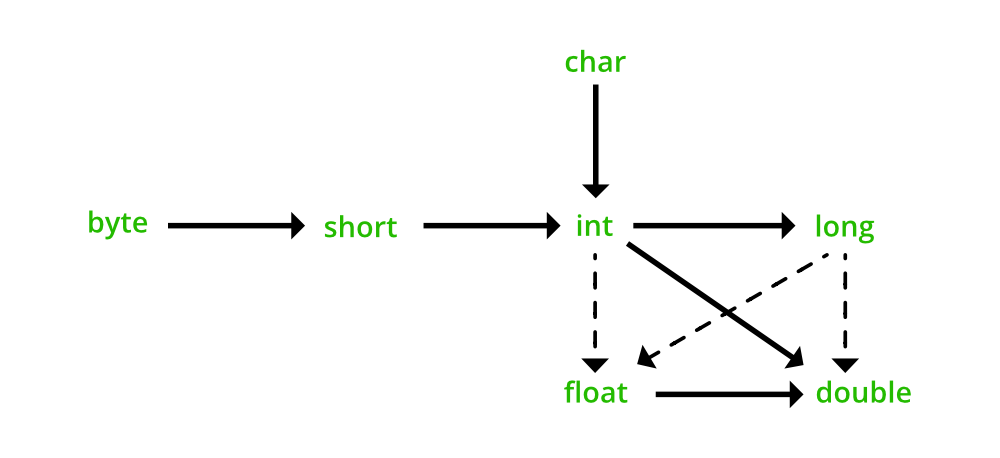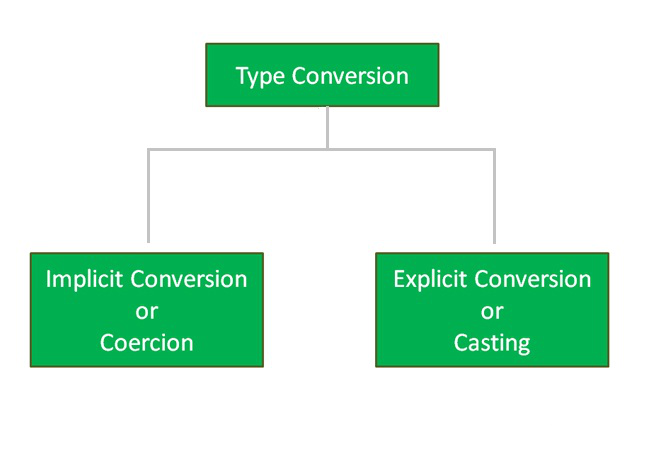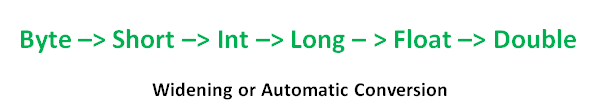# Coercion in Java

• Difficulty Level : Easy
• Last Updated : 25 Feb, 2022

Conversion of one data type to another is nowadays easy as many languages including Java supports the conversion as a convenience to the programmer. Here conversion can be done in two ways i.e. implicit or explicit conversion.• implicit conversion: This type of conversion is automatically done by the programming languages. In the case of Java, it is done by the JVM ( Java Virtual Machine ).
• explicit conversion: This type of conversion has to be done by the programmer as per their requirements. (No rule of JVM)

## Coercion in Java

here we will be discussing coercion (also known as type conversion). So, Coercion is the process of converting one type of data type to another. In a simple way, implicit conversion holds the title of coercion in java.Example 1

## Java

 `// Java Program to Illustrate Coercion Via` `// Integer  Data Type to Double  Data Type`   `// Importing required classes` `import` `java.io.*;`   `// Class` `class` `GFG {`   `    ``// Main driver method` `    ``public` `static` `void` `main(String[] args)` `    ``{`   `        ``// Declaring and initializing` `        ``// integer and double variables` `        ``int` `numerator = ``15``;` `        ``double` `result = numerator / ``5``;`   `        ``// Print statement` `        ``System.out.println(``"Result is in double format : "` `                           ``+ result);` `    ``}` `}`

Output

`Result is in double format : 3.0`

In the above example, the constant (numerator) 15 is an integer but its context requires a double value.

Output explanation: Machine operation is performed on data at the execution time of the program are:

• Fetches the value of the numerator variable.
• Use of the literal value i.e. 5.
• Converts the value of the literal value to a floating point.
• Performs floating-point division (CPU task).
• Stores the result into memory allocated to the result variable.

Example 2

## Java

 `// Java Program to Illustrate Coercion Via` `// Character data type to Integer data type`   `// Importing required classes` `import` `java.io.*;`   `// Class` `class` `GFG {`   `    ``// Main driver method` `    ``public` `static` `void` `main(String[] args)` `    ``{`   `        ``// Declaring character and integer variables` `        ``char` `alphabet = ``'A'``;` `        ``int` `ascii_value = alphabet;`   `        ``// Print statement` `        ``System.out.println(``"ASCII Value of A is: "` `                           ``+ ascii_value);` `    ``}` `}`

Output

`ASCII Value of A is: 65`

In the above example, the ascii_value is an integer but its context requires a character value therefore with the help of coercion/implicit conversion it is possible with ease.

Here are a few more examples of few coercion done by JVM as listed below as follows:

• byte + short = int
• short + byte = int
• char + short = int
• byte + byte = int
• int + int = int
• int + float = float
• long + float = float
• float + double = double
• long + double = double….. and many more.

Note: In the above points ” + ” be any arithmetic operator which is suitable with the corresponding data types.Casting is the process by which we treat an object type as another type while coercing is the process of converting one object to another. There are two ways to cast the primitive data type, widening, and narrowing. It is also known as implicit type casting because it is done automatically.

My Personal Notes arrow_drop_up
Recommended Articles
Page :